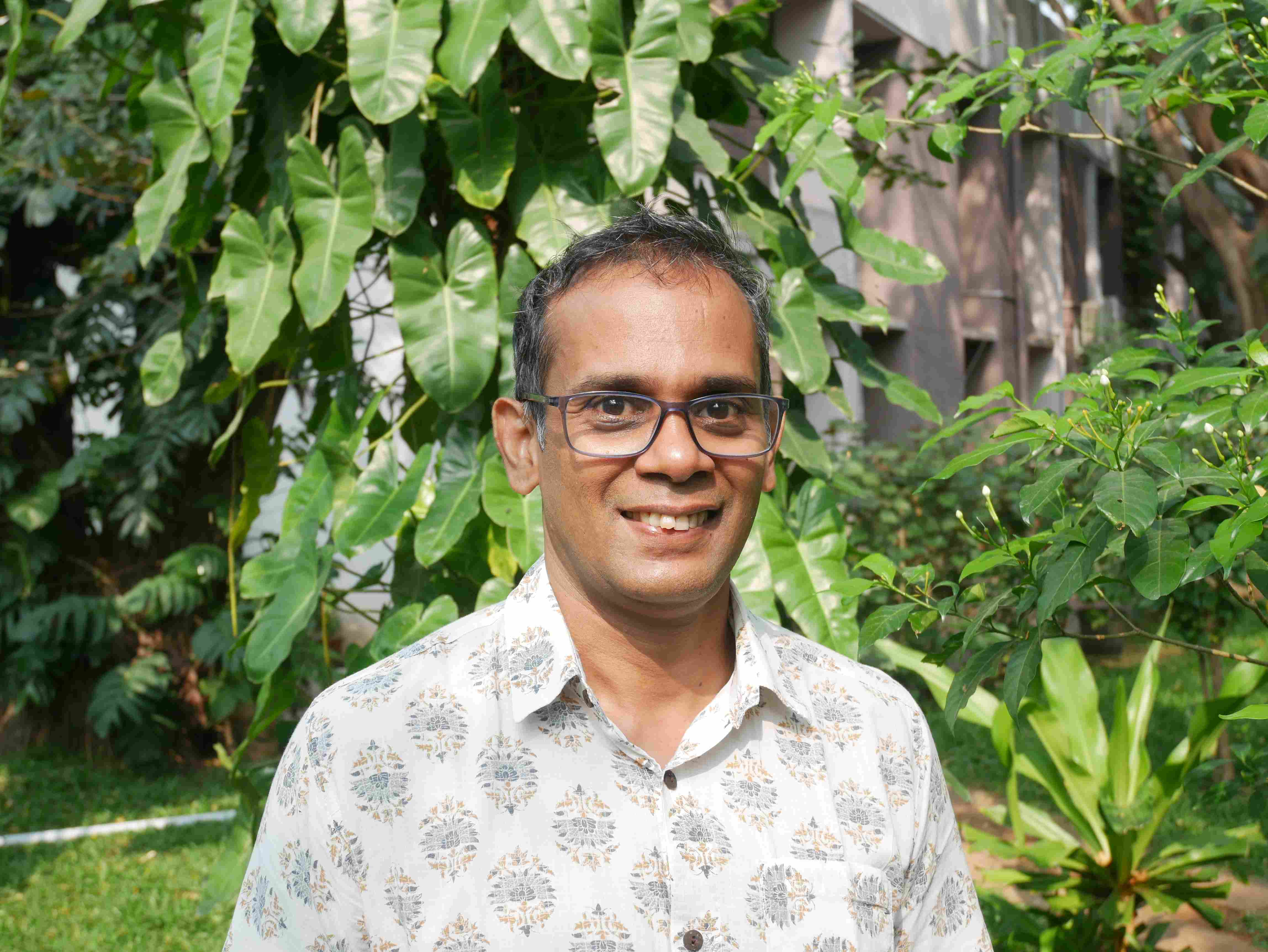Professor

amri@imsc.res.in

+91 44 2254 3207

Areas of Interest

Representation theory

Combinatorics

## Recent Work

##### On the Existence of Elementwise Invariant Vectors in Representations of Symmetric Groups
###### with Amrutha P and Velmurugan S

We determine when a permutation with cycle type $\mu$ admits a non-zero invariant vector in the irreducible representation $V_\lambda$ of the symmetric group. We find that a majority of pairs $(\lambda,\mu)$ have this property, with only a few simple exceptions.

##### Representation zeta functions of arithmetic groups of type $A_2$ in positive characteristic
###### with Uri Onn and Pooja Singla

We prove two conjectures regarding the representation growth of groups of type $A_2$. The first, conjectured by Avni, Klopsch, Onn and Voll, regards the uniformity of representation zeta functions over local complete discrete valuation rings. The second is the Larsen--Lubotzky conjecture on the representation growth of irreducible lattices in groups of type $A_2$ in positive characteristic assuming Serre's conjecture on the congruence subgroup problem.

##### Enumeration of Anti-Invariant Subspaces and the q-Hermite Catalan Triangle
###### with Samrith Ram

We express the number of anti-invariant subspaces for a linear operator on a finite vector space in terms of the number of its invariant subspaces. When the operator is diagonalizable with distinct eigenvalues, our formula gives a finite-field interpretation for the entries of the $q$-Hermite Catalan matrix. We also obtain an interesting new proof of Touchard's formula for these entries.